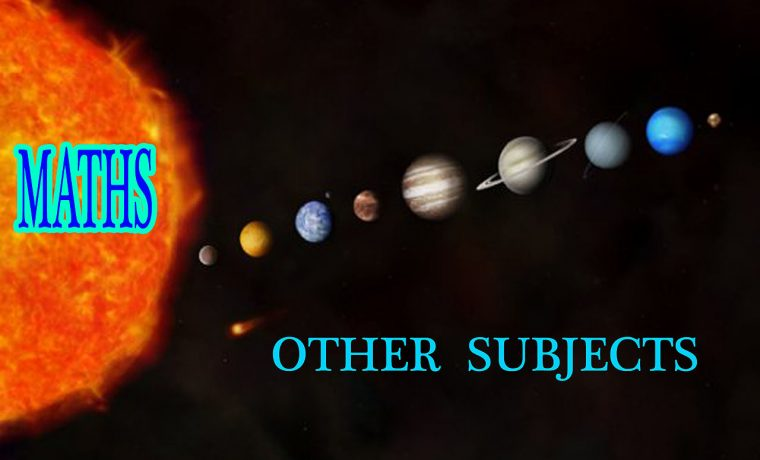# How is Mathematics Related to Other Subjects?

Mathematics is a subject that is intimately connected to all disciplines like science, engineering, arts, statistics, literature etc. It is an integral part of human thought and logic. It gives an efficient way of developing mental discipline and it promotes logical thinking. Mathematics plays a significant role in understanding the content of other subjects. Let us discuss in detail about how mathematics is related to other subjects.

## Science

Without mathematics, science is totally meaningless. Because, all the scientific theories, chemical reactions are only counted with the help of mathematics.

• In physics, to understand any concepts like force, laws of motion, gravitation, pressure etc., mathematical knowledge is a must.
• In chemistry, understanding of maths concepts helps to calculate the electronic configuration of atom, empirical and molecular formula, balancing chemical equations and so on.
• In Biology, it has a high correlation as it involves the calculation of respiration rate, caloric value, measure blood pressure, counting of RBC and WBC, measuring the circumference of a circle of a cell.

## Engineering

Engineering has a strong correlation with mathematics. It is used in all the branches of engineering like Computer Science, Electronics, Civil, Electrical, Mechanical etc. All the Software products, electrical circuits, electronic products are operated only with the help of mathematical information.

## Literature

In literature, logical thinking used in mathematics helps to write the poem clearly and logically. It helps authors to present a poem with a mathematical structure like a number of words in a line, a number of lines in a stanza and so on. It provides the shape of a poem.

## Statistics

In statistics, mathematical methods are used to calculate the economic conditions such as import and export trends, economic forecasting and study of different distributions like beta distribution, probability distribution etc. It helps in calculating various indexes like production inflation, deflation etc.

## Arts

Mathematics links music to numbers because music is a pattern. Every rhythm and scale is a pattern. Pythagoras used numerical terms to express the interval between notes from the geometrical pattern and it indicates the length of notes.

Subscribe to BYJU’S YouTube Channel to learn more concepts with from Maths and Science and explore more videos.